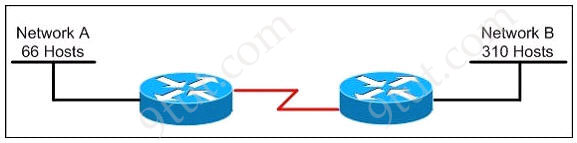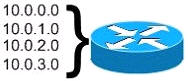# » CCNA – Subnetting Questions 2

Ensurepass
Question 1

Refer to the exhibit. Which VLSM mask will allow for the appropriate number of host addresses for Network A?A. /25
B. /26
C. /27
D. /28

Explanation

We need 66 hosts < 128 = 27 -> We need 7 bits 0 -> The subnet mask should be 1111 1111.1111 1111.1111 1111.1000 0000 -> /25

Question 2

Refer to the exhibit. Which subnet mask will place all hosts on Network B in the same subnet with the least amount of wasted addresses?A. 255.255.255.0
B. 255.255.254.0
C. 255.255.252.0
D. 255.255.248.0

Explanation

310 hosts < 512 = 29 -> We need a subnet mask of 9 bits 0 -> 1111 1111.1111 1111.1111 1110.0000 0000 -> 255.255.254.0

Question 3

Refer to the exhibit. Which mask is correct to use for the WAN link between the routers that will provide connectivity while wasting the least amount of addresses?A. /23
B. /24
C. /25
D. /30

Explanation

For WAN link we only need 2 usable host addresses for 2 interfaces on the routers. The subnet mask of /30 gives us 22 – 2 = 2 usable host addresses. Also remember that “/30″ is famous for point-to-point connection because it wastes the least amount of addresses.

Question 4

Refer to the exhibit. What is the most appropriate summarization for these routes?A. 10.0.0.0/21
B. 10.0.0.0/22
C. 10.0.0.0/23
D. 10.0.0.0/24

Explanation

We need to summarize 4 subnets so we have to move left 2 bits (22 = 4). In this question we can guess the initial subnet mask is /24 because 10.0.0.0, 10.0.1.0, 10.0.2.0, 10.0.3.0 belong to different networks. So “/24″ moves left 2 bits -> /22.

Question 5

On the network 131.1.123.0/27, what is the last IP address that can be assigned to a host?

A. 131.1.123.30
B. 131.1.123.31
C. 131.1.123.32
D. 131.1.123.33

Explanation

Increment: 32

Both 131.1.123.30 & 131.1.123.33 can be assigned to host but the question asks about the “last IP address” so A is the correct answer.

Question 6

The ip subnet zero command is not configured on a router. What would be the IP address of Ethernet0/0 using the first available address from the sixth subnet of the network 192.168.8.0/29?

A. 192.168.8.25
B. 192.168.8.41
C. 192.168.8.49
D. 192.168.8.113

Explanation

The “ip subnet zero” is not configured so the first subnet will start at 192.168.8.8 (ignoring 192.168.8.0).

Increment: 8
1st subnet: 192.168.8.8
2nd subnet: 192.168.8.16
3rd subnet: 192.168.8.24
4th subnet: 192.168.8.32
5th subnet: 192.168.8.40
6th subnet: 192.168.8.48 -> The first usable IP address of 6th subnet is 192.168.8.49

Question 7

For the network 192.0.2.0/23, which option is a valid IP address that can be assigned to a host?

A. 192.0.2.0
B. 192.0.2.255
C. 192.0.3.255
D. 192.0.4.0

Explanation

Increment: 2

Question 8

How many addresses for hosts will the network 124.12.4.0/22 provide?

A. 510
B. 1022
C. 1024
D. 2048

Explanation

/22 gives us 10 bits 0 -> 210 – 2 = 1022. Notice that the formula to calculate the number of host is: 2k – 2.

Question 9

The network default gateway applying to a host by DHCP is 192.168.5.33/28. Which option is the valid IP address of this host?

A. 192.168.5.55
B. 192.168.5.47
C. 192.168.5.40
D. 192.168.5.32
E. 192.168.5.14

Question 10

Which two addresses can be assigned to a host with a subnet mask of 255.255.254.0? (Choose two)

A. 113.10.4.0
B. 186.54.3.0
C. 175.33.3.255
D. 26.35.2.255
E. 17.35.36.0